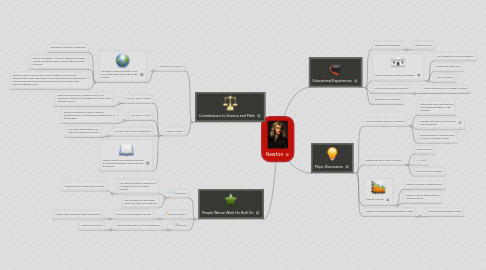# Newton

Solve your problems or get new ideas with basic brainstorming

Get Started. It's FreeNewton## 1. Educational Experiences

### 1.1. Raised by gransparents

1.1.1. Sent to school

### 1.2. sent to grammar school in Grantham

1.2.1. Not interested in normal subjects

1.2.2. Reads and thinks a lot

1.2.3. Top of his class

### 1.3. Attends Cambridge University

1.3.1. Studies Aristotle and the works of many

## 2. Major Discoveries

### 2.1. Force of gravity works at a distance

2.1.1. Nature has basic laws that are the same everywhere in the Universe

2.1.2. Explains the motion of the moon and the planets

2.1.3. Describes how to find the mass of the Sun and the planets

### 2.2. Establishes the 3 laws of motion

2.2.1. Law of Inertia

2.2.2. F = m(a)

2.2.3. Law of Action-Reaction

### 2.3. Invents Calculus

2.3.1. Describes waves mathematically

2.3.2. Explains how to determine the speed of sound

### 2.4. Makes discoveries about the nature of light

2.4.1. Determines the speed of light

## 3. People Whose Work He Built On

### 3.1. Descartes

3.1.1. The natural motion of objects is in a straight line at a constant velocity

3.1.1.1. Newton produces the idea of inertia

3.1.2. The Universe can be broken down into parts and analyzed

### 3.2. Edmond Halley

3.2.1. Wants to prove Kepler's 3rd law

3.2.1.1. Newton says planetary orbits are elliptical

### 3.3. Roener

3.3.1. Believes that light is not instantaneous

3.3.1.1. Newton proves this

## 4. Contributions to Science and Math

### 4.1. Invention of Calculus

4.1.1. Develops Universal Gravity = the force that keeps your feet on the ground

4.1.1.1. The Law of Universal Gravitation

4.1.1.2. Idea of Gravitation = force of attraction between masses no matter what or where they are in the Universe

4.1.1.3. Creates Inverse Square Law = every object in the Universe attracts every other object with a force proportional to the product of the masses and inversely proportional to the square of the distance between them

### 4.2. Laws of Motion

4.2.1. 1st Law: Law of Inertia

4.2.1.1. Every body remains in a state of rest, or of unchanging motion in a straight line unless acted upon by a force

4.2.2. 2nd Law: F = m(a)

4.2.2.1. The force acting on a body is directly proportional to, and in the same direction as, its acceleration

4.2.3. 3rd Law: Law of Action-Reaction

4.2.3.1. For every action there is an equal and opposite reaction

4.2.4. Newton wrote "Philosophiae Naturalis Principia Mathematica" describing his discoveries